Most Affordable JEE | NEET | 8,9,10 Preparation by Kota's Top IITian Doctor Faculties

# Classification of Elements and Periodicity in Properties Important Questions & Answers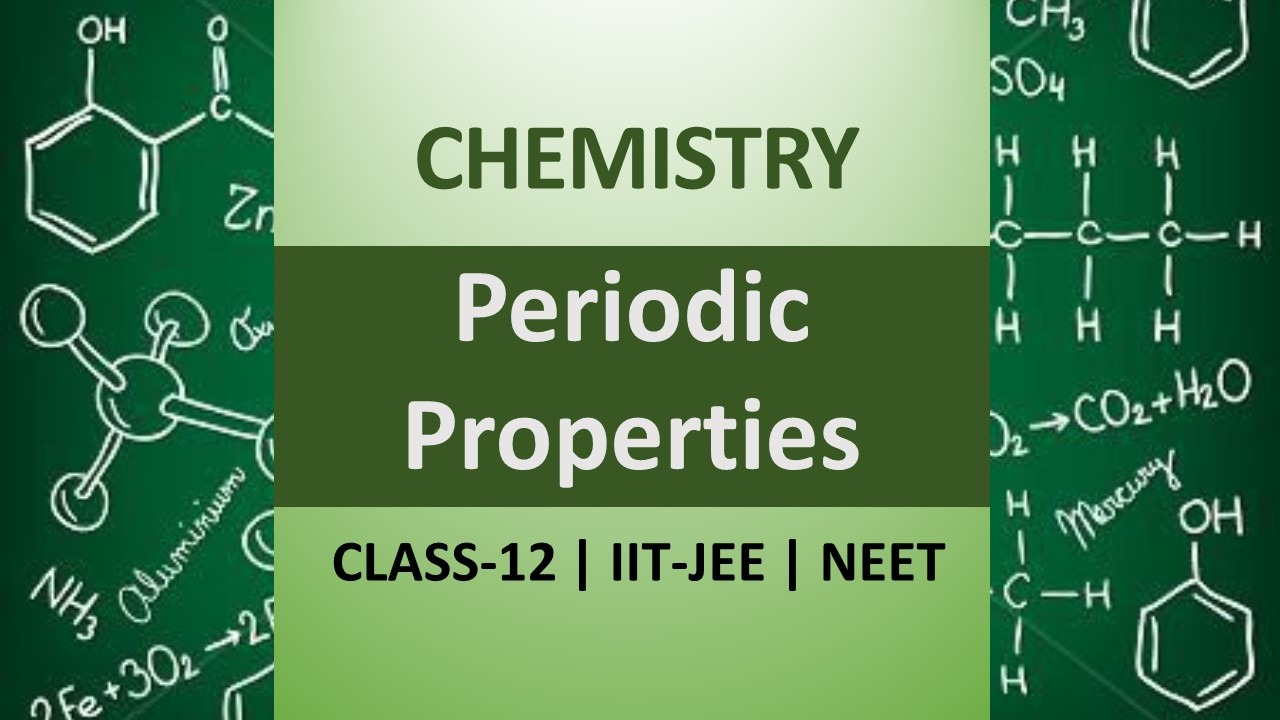`
Get Classification of Elements and Periodicity in Properties chapter's important questions & answers. View the Important Question bank for Class 11 Chemistry. These important questions will play significant role in clearing concepts of Chemistry. This question bank is designed keeping NCERT in mind and the questions are updated with respect to upcoming Board exams. You will get here all the important questions for class 11 chemistry chapters. Click Here for Detailed Chapter-wise Notes of Chemistry for Class 11th, JEE & NEET.  You can access free study material for all three subject’s Physics, Chemistry and Mathematics. Click Here for Detailed Notes of any chapter.  eSaral provides you complete edge to prepare for Board and Competitive Exams like JEE, NEET, BITSAT, etc. We have transformed classroom in such a way that a student can study anytime anywhere. With the help of AI we have made the learning Personalized, adaptive and accessible for each and every one. Visit eSaral Website to download or view free study material for JEE & NEET. Also get to know about the strategies to Crack Exam in limited time period.
Q. State modern periodic law. [NCERT]
Ans. The properties of elements are periodic function of their atomic number.
Q. Why do elements in the same group have similar physical and chemical properties ? [NCERT ]
Ans. Because they have similar valence shell electronic configuration.
Q. In terms of period and group where would you locate the element with Z = 14 ? [NCERT ]
Ans. Period – 3, Group – 14.
Q. In the modern periodic table, the period indicates the value of (i) Atomic number (ii) Mass number (iii) Principal quantum number (iv) Azimuthal quantum number. [NCERT]
Ans. In the modern periodic table, each period begins with the filling with a new shell, therefore, the period indicates the value of principal quantum number. Thus option (iii) is correct.
Q. What would be the IUPAC name and symbol of the element with atomic number 120 ? [NCERT]
Ans. The roots for $1,2$ and 0 are $u n, b i,$ and nil respectively. $\therefore$ Name of element: Unbinilium Symbol : Ubn.
Q. Which element do you think would have been named by (i) Lawrence Berkalay Laboratory (ii) Seaborg’s group? [NCERT]
Ans. (i) Lawrencium (Z=103) and Berkelium (Z=97). (ii) Seaborgium (Z=106)
Q. How would you react to the statement that electronegativity of on Pauling scale is 3.0 in all its compounds ? [NCERT]
Ans. The electronegativity of nitrogen will not be 3.0 in all its compounds. It depends upon other atom attached to it.
Q. Arrange the following ions in the order of increasing size: $B e^{2+}, C l^{-}, S^{2}, N a^{+}, M g^{2+}, B r^{-}$ [NCERT]
Ans. $\mathrm{Be}^{-2}, \mathrm{Mg}^{-2}, \mathrm{Na}^{+}, \mathrm{Cl}^{-}, \mathrm{s}^{-2}, \mathrm{Br}^{-}$
Q. Would you expect the first ionization enthalpies for two isotopes of the same element to be the same or different ? Justify your answer. [NCERT]
Ans. Isotopes are atoms of the same element which have same atomic number but different mass number. Therefore, they have same number of electrons and nuclear charge (protons). Thus, they will have same first ionization enthalpies.
Q. What do you understand by isoelectronic species ? Give the formula of a species that will be isoelectronic with the following atoms or ions :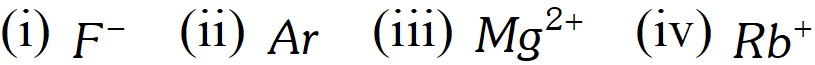[NCERT]
Ans. Isoelectronic species are those which have same number of electrons. (i) $F^{-}\left(10 e^{-}\right): O^{2-}$ (ii) $A r\left(18 e^{-}\right): C l^{-}$ (iii) $M g^{2+}\left(10 e^{-}\right): N a^{+}$ (iv) $R b^{+}\left(36 e^{-}\right): K r$
Q. Are the oxidation state and covalency of $A l$ in $\left[A I C I\left(H_{2} O\right)_{5}\right]^{2+}$ same ? [NCERT]
Ans. - No, the oxidation state of $A l$ is $+3$ and covalency is 6
Q. Show by a chemical reaction with water that $N a_{2} O$ is basic oxide and $C l_{2} O_{7}$ is an acidic oxide. [NCERT]
Ans.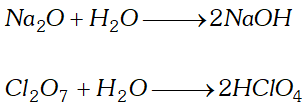Q. What is the basic theme of organization of elements in the periodic table. (NCERT)
Ans. The basic theme of organisation of elements in the periodic table is to simplify and systematize the study of the properties of all the elements and millions of their compounds. On the basis of similarities in chemical properties, the various elements has now been divided into different groups. This has made the study simple because the properties of elements are now studied in form of groups rather than individually.
Q. Write the atomic number of the element in the third period and seventeenth group of the periodic table. [NCERT]
Ans. In the third period the filling up of only 3s- and 3p- orbitals occur. Therefore, in this period there are only two s- and six p-block elements. Since third period starts with and ends at , therefore, elements with and are s- block elements. The next six elements with to 18 are p- block elements and belong to groups 13, 14, 15, 16, 17 and 18. Therefore, the elements which will lie in seventeenth group will have
Q. In terms of period and group where would you locate the element with Z = 114 ? So, it belongs to $7^{\text {th }}$ period and $14^{\text {th }}$ group. [NCERT]
Ans. The electronic configuration of the element with $Z=114$ would be $[R n\} 5 f^{14} 6 d^{10} 7 s^{2} 7 p^{2} .$ since it has $n=7$ for the valence shell, it belong to the $7^{\text {th }}$ period. It receives the last electron in $p$ -orbital. therefore, it belongs to $p$ -block. The group number will be $10+4$ (No. of electrons in $n s \text { and } n p \text { orbitals })=14$ So, it belongs to $7^{\text {th }}$ period and $14^{\text {th }}$ group.
Q. How would you justify that there are only 18 elements in the fifth period of the periodic table. [NCERT ]
Ans. The fifth period beings with the filling of 5s orbital and continues till the filling of sixth energy level (6s) starts. The sub-shell which follow 5s are .Thus, the elements which involve filling of 5s, 4d and 5p sub-shell are accommodated in the fifth period. These sub-shells have nine orbitals that can accept 18 electrons in all. Hence, there are 18 elements in the fifth period.
Q. On the basis of quantum numbers, justify that the sixth period of the periodic table should have 32 elements. [NCERT ]
Ans. In the sixth period the subshell filled are and . There is a total of 16 orbitals which can accommodate 32 electrons. Hence there are 32 elements in the sixth period.
Q. Consider the following species : $N^{3-}, O^{2-}, F^{-}, N a^{+}, M g^{2+}$ and $A l^{3+}$ (i) What is common in them ? (ii) Arrange them in the order of increasing ionic radii. [NCERT]
Ans. (i) All these ions have same number (10) of electrons. Therefore, there are also called isoelectronic species. (ii) Since the number of electrons are same, the ionic size decrease with increase in nuclear charge. Therefore, the ions can be arranged in increasing order of ionic radii as $A l^{3+} Q. Anything that influences the valence electrons will affect the chemistry of the element. Which one of the following factors does not affect the valence shell (i) Valence principal quantum number (ii) Nuclear charge (Z) (iii) Nuclear mass (iv) Number of core electrons. [NCERT] Ans. Nuclear mass does not affect the valence shell because nucleus consists of protons and neutrons. Where as protons i.e., nuclear charge affects the valence shell but neutrons do not. Thus option (iii) is wrong. Q. Which of the following statements related to the modern periodic table is incorrect ? (i) The p-block has six columns, because a maximum of 6 electrons can occupy all the orbitals in a p-subshell. (ii) The d-block has 8 columns, because a maximum of 8 electrons can occupy all the orbitals in a d-subshell. [NCERT] Ans. Statement (ii) is incorrect while other statements are correct. The correct statement (ii) is : the d-block has 10 columns, Statement (ii) is incorrect while other statements are correct. The correct statement (ii) is : the d-block has 10 columns, Q. What is the basic difference in approach between Mendeleev’s Periodic Law and the Modern Periodic Law? [NCERT] Ans. Mendeleev Periodic Law states that the physical and chemical properties of the elements are a periodic function of their atomic weights while Modern Periodic Law states that physical and chemical properties of elements are a periodic function of their atomic number. Thus, the basic difference in approach between Mendeleev’s Periodic Law and Modern Periodic Law is the change in basis of classification of elements from atomic weight to atomic number. Q. How do atomic radii vary in a period and in a group ? How do you explain the variation ? [NCERT] Ans. Within a group, the atomic radius increases down the group. This is because a new energy shell (i.e., principal quantum number increases by unity) is added at each succeeding element while the number of electrons in the valence shell remains to be the same. In other words, the electrons in the valence shell of each succeeding element lie farther and farther away from the nucleus. As a result, the force of attraction of the nucleus for the valence electrons decreases and hence the atomic size increases. In contract, the atomic size decreases as we move from left to right in a period. This is because that within a period the outer electrons remains in the same shell but the nuclear charge increases by one unit at each succeeding element. Due to this increased nuclear charge, the attraction of the nucleus for the outer electrons increases and hence the atomic size decreases. Q. Considering the atomic number and position in the periodic table, arrange the following elements in the increasing order of metallic character. : Si, Be, Mg, Na, P. [NCERT] Ans. Arranging the elements into different groups and periods in order of their increasing atomic numbers, we have,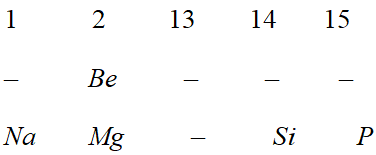We know that the metallic character increases down a group and decreases along a period as we move from left to right. Therefore, Na is the most metallic element, followed by Mg and Si, while P is the least metallic element. Among and is more metallic than Be. Therefore, the overall increasing order of metallic character is$P
Q. Write the general electronic configuration of s-, p-, d- and f-block elements : [NCERT]
Ans. (i) $s$ -Block elements: $n s^{1-2}$ where $n=2-7$ (ii) $\quad p$ -Block elements: $n s^{2} n p^{1-6}$ where $n=2-6$ (iii) $d$ -Block elements: $(n-1) d^{1-10} n s^{0-2}$ where $n=4-7$ (iv) $f$ -Block elements: $(n-2) f^{0-14}(n-1) d^{0-1} n s^{2}$ where $n=6-7$
Q. Assign the position of the element having outer electronic configuration, (i) $n s^{2} n p^{4}$ for $n=3 \quad$ (ii) $(n-1) d^{2} n s^{2}$ for $n=4$ and (iii) $(n-2) f^{7}(n-1) d^{1} n s^{2}$ for $n=6$ in the periodic table. [NCERT]
Ans. (i) $\quad n=3$ suggest that the elements belongs to third period. since the last electron enters the $p$ -orbital, therefore, the given element is a $p$ -block element. Further since the valence shell contains $6(2+4)$ electrons, therefore, group number of the elements $=10+$ no. of electrons in the valence shell $=10+6=16$ The complete electronic configuration of the element is $1 s^{2} 2 s^{2} 2 p^{6} 3 s^{2} 3 p^{4}$ and the element is $S($ sulphur). (ii) $\quad n=4$ suggest that the element lies in the $4^{\text {th }}$ Period. since the $d$ -orbitals are incomplete, therefore, it is $d$ -block element. The group number of the element $=$ no. of $d$ -electrons $+$ no. of $s$ electrons $=2+2=4 .$ Thus the elements lies in group 4 and $4^{\text {th }}$ period. The complete electronic configuration of the element is $1 s^{2} 2 s^{2} 2 p^{6} 3 s^{2} 3 p^{6} 3 d^{2} 4 s^{2}$ and the element in Ti (Titanium). (iii) $\quad n=6$ means that the element lies in the sixth period. since the last electron goes to the $f$ -orbital, therefore, the element is a $f$ -block element. All $f$ -block elements lie in group 3. The complete electronic configuration of the element is $[\mathrm{Xe}\} 4 f^{7} 5 d^{1} 6 s^{2} .$ The atomic number of the element $54+7+1+2=64$ and the element $G d($ gadolinium).
Q. The elements Z = 117 and 120 have not yet been discovered. In which family group would you place these elements and also give electronic configuration in each case [NCERT]
Ans. The electronic configuration of the element with $Z=117$ would be $[R n] 4 f^{14} 5 d^{10} 7 s^{2} 7 p^{5} .$ It has outermost $n s^{2} n p^{5}$ configuration and therefore, it belongs to halogen family or group 17 The electronic configuration of the element with $Z=120$ would be [Uuo] $8 s^{2} .$ It has outermost $n s^{2}$ configuration and therefore, belongs to alkaline earth metals family or group 2.
Q. What are major differences between metals and non-metals? [NCERT]
Ans. Elements which have a strong tendency to lose electrons to form cations are called metals while those which have a strong tendency to accept electrons to form anions are called non metals. Thus, metals are strong reducing agents, they have low ionization enthalpies, have less negative electron gain enthalpies, low electronegativity, form basic oxides and ionic compounds. Non-metals, on the other hand, are strong oxidising agents, they have high ionization enthalpies, have high negative electron gain enthalpies, high electronegativity, form acidic oxides and covalent compounds.
Q. The elements Z=107 and Z=109 have been made recently; element Z=108 has not yet been made. Indicate the group in which you will place the above elements. [NCERT]
Ans. The electronic configuration of these elements are : $Z=107 \quad[R n] 5 f^{14} 6 d^{5} 7 s^{2}$ $Z=108 \quad[R n] 5 f^{14} 6 d^{6} 7 s^{2}$ $Z=109 \quad[R b] 5 f^{14} 6 d^{7} 7 s^{2}$ These elements will be placed in $d$ -block in group $7^{\text {th }}, 8^{\text {th }}$ and $9^{\text {th }}$ respectively.
Q. Which of the elements $N a, M g, S i$ and p would have the greater difference between the first and the second ionization enthalpies ? Briefly explain your answer. [NCERT]
Ans. - Among these elements, $N a$ is an alkali metal and has only one electron in its valence shell $\left(3 s^{1}\right) .$ Therefore, its $I E_{1}$ is very low. After the removal of one electron, it acquires neon configuration $i . e ., \quad\left(1 s^{2} 2 s^{2} 2 p^{6}\right) .$ Therefore, its $I E_{2}$ is expected to be very high. Consequently the difference in first and second ionisation enthalpies would be greater in case of $N a$
Q. Energy of an electron in the ground state of the hydrogen atom is $-2.18 \times 10^{-18} \mathrm{J}$. Calculate the ionization enthalpy of atomic hydrogen in terms of $J$ mol $^{-1}$
Ans. Energy of the electron in ground state $=-2.18 \times 10^{-18} \mathrm{J}$ Energy required for removal of the electron $=0-\left(-2.18 \times 10^{-18} \mathrm{J}\right)=2.18 \times 10^{-18} \mathrm{J}$ This is the energy required to remove the electron from one atom of hydrogen in ground state. The energy required to remove electron from one mole $\left(6.022 \times 10^{23} \text { atoms }\right)$ will be $=2.18 \times 10^{-18} \times 6.022 \times 10^{23}=13.12 \times 10^{5} \mathrm{J} \mathrm{mol}^{-1}$
Q. Arrange the following elements in the increasing order of metallic character : Si, Be, Mg, Na, P. [NCERT]
Ans. We know that the metallic character increases down a group and decreases along a period as we move from left to right. Hence the order of increasing metallic character is $P Q. (i) Why do Group-I metals have lower ionisation enthalpy than corresponding Group-II metals? (ii) Why is an anion larger in size than its neutral atoms? Ans. (i) Group I elements are largest in size than alkaline earth metals (Group II elements), therefore, There is less force of attraction between nucleus and valence electron, that is why their ionization energy is lower. (ii) Anions are largest than neutral atom because electrons are more than the protons, therefore, effective nuclear charge is less, therefore, distance between centre of nucleus and valence electrons is more. Q. The first ionization enthalpy$\left(\Delta_{i} H\right)$values of the third period elements,$N a, M g,$and$S i$are respectively$496,737$and$786 \mathrm{kJ} \mathrm{mol}^{-1} .$Predict whether the first$\Delta_{i} H$value for$A l$will be more close to 575 or$760 \mathrm{kJ} \mathrm{mol}^{-1}$respectively. Justify your answer. [NCERT] Ans. It will be more close to$575 \mathrm{kJ} \mathrm{mol}^{-1}$because the value of Al should be lower than that of$M g .$This is due to effective shielding of$3 p$electrons from the nucleus by$3 s$-electrons. Q. Explain why cations are smaller and anions are larger in radii than their parent atoms. [NCERT] Ans. The ionic radius of a cation is always smaller than the parent atom because the loss of one or more electrons increases the effective nuclear charge. As a result, the force of attraction of nucleus for the electrons increases and hence the ionic radii decreases. In contrast, the ionic radius of an anion is always larger than its parent atom because the addition of one or more electrons decreases the effective nuclear charge. As a result, the force of attraction of the nucleus for the electrons decreases and hence the ionic radii increases. Q. What is the basic difference between the terms electron gain enthalpy and electronegativity? [NCERT] Ans. Both electrons gain enthalpy and electronegativity refer to the tendency of the atom of an element to attract electrons. Whereas electron gain enthalpy refers to the tendency of an isolated gaseous atom to accept an additional electron to form a negative ion, electronegativity refers to the tendency of the atom of an element to attract the shared pair of electrons towards it in a covalent bond. Q. (i) Predict the position of the element with atomic number 26 in the periodic table. (ii) Why IE of oxygen is less than that of nitrogen ? Ans. (i) It belong to group 8 of periodic table because its electronic configuration is$[\mathrm{Ar}] 4 \mathrm{s}^{2} 3 d^{6}$(ii) It is because nitrogen has stable electronic configuration i.e., half-filled$p$-orbitals$\therefore$its I.E. is more than that of oxygen. Q. The first ionization enthalpy$\left(\Delta_{i} H\right)$values of the third period elements,$\mathrm{Na}, \mathrm{Mg}$and$\mathrm{Si}$are respectively$496,737$and 786 kJ mol$^{-1}$predict whether the$\Delta i H$value for$A l$will be more close to 575 or$760 \mathrm{kJ} \mathrm{mol}^{-1}$. Justify your answer. [NCERT] Ans. Arrange the elements$N a, M g$and$S i$into different groups and periods in order of their increasing atomic numbers, we have,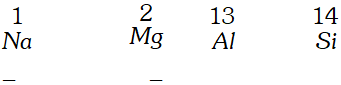since in case of$A l,$a$3 p$-electron is to be lost while in$M g,$a$2 s$electron is to be lost, therefore, value for$A l$will be lower than that of$M g\left(737 k J \mathrm{mol}^{-1}\right)$because of the effective shielding of the$3 p-$electron from the nucleus by 3 s-electrons. Therefore,$\Delta_{i} H$for$A l$will be more close to$575 \mathrm{kJ} \mathrm{mol}^{-1}$Q. The size of isoelectronic species$-F^{-}, N e$and$N a^{+}$is affected by (i) Nuclear charge$(Z)$(ii) Valence principal quantum number$(n)$(iii) Electron-electron interaction in the outer orbital (iv) None of factors because their size is the same. [NCERT] Ans. - The size of the isoelectronic ions depends upon the nuclear charge$(Z) .$As the nuclear charge increase the size decreases. For example,$F^{-}(+9)>N e(+10)>N a^{+}(+11) .$Therefore, statement (i) is correct while all other statements are wrong. Q. The increasing order of reactivity among group 1 elements is$L iC D>B r>$I. Explain. [NCERT] Ans. The elements of group 1 have only one electron in their respective valence shell and thus a strong tendency to lose this electron. The tendency to lose electrons. in turn, depends upon the ionization enthalpy. Since the ionization enthalpy decreases down the group, therefore, the reactivity of group 1 elements increases in the same order :$L iC l(+1.36 V), B r(1.08 V)$and$I(+0.53),$therefore, their reactivities also decrease in the same order:$F>C l>B r>I$Q. Which of the following species has the largest and the smallest size.$M g, M g^{2+}, A l, A l^{3+}$[NCERT] Ans. (i)$\quad M g$and$A l$belong the third period. Across a period, atomic radii decreases due to increased nuclear charge. Therefore, atomic size of Al is smaller than that of$M g .$(ii) Further, cations are smaller than their parent atoms. Therefore,$M g^{2+}$is smaller than$M g$and$A l^{3+}$is smaller than$A l$(iii) and are isoelectronic ions. Among isoelectronic ions, higher the tye charge, smaller the size. Therefore, ionic radius of$A P^{3+}$is smaller than that of From the above discussion, it follows that$M g$has the largest while has the smallest size. Q. What is the significance of the terms ‘isolated gaseous atom’ and ‘ground state’ while defining the ionization enthalpy and electron gain enthalpy ? Ans. (i) Ionization enthalpy is the minimum amount of energy required to move the most loosely bound electrons from an isolated gaseous atom so as to convert it into a gaseous cation is called ionization enthalpy. The force with which an electron is attracted by the nucleus of an atom is appreciably affected by presence of other atoms within its molecule or in the neighbourhood. Therefore, for the purpose of determination of ionization enthalpy, it is essential that these interatomic forces of attraction should be minimum. Since in the gaseous state, the atoms are widely separated, therefore, these interatomic forces are minimum. Further since it is not possible to isolate a single atom for the purpose of determination of its ionization enthalpy, therefore, the interatomic distances are further reduced by carrying out the measurement at a low pressure of the gaseous atom. Due to these reasons, the term isolated gaseous atoms has been included in the definition of ionization enthalpy. (ii) Electron gain enthalpy is the energy released when an isolated gaseous atom in the ground state accepts an extra electron to form the gaseous negative ion. The term ground state here means that the atom must be present in the most stable state, i.e., the ground state. The reason being that when the isolated gaseous atoms is in the excited state, lesser amount of energy will be released when it gets converted into gaseous anions after the accepting an electron. Therefore, for comparison purposes, the electron gains enthalpy of gaseous atoms be determined in their respective most stable state, i.e., ground state. Q. (i) Write the electronic configurations of the elements given below:$A(\text { At. } \mathrm{No} .=9), B(\text { At. } \mathrm{No} .=12), C \quad(\text { At. } \mathrm{No} .=29), D(\mathrm{At}\mathrm{No} .=54), E(\mathrm{At.} \mathrm{No} .=58)$(ii) Also predict the period, group number and block to which they belong. (iii) Classify them as representative elements, noble gases, transition and inner transition elements. Ans. (i) Electronic configuration of elements A, B, C, D and E are as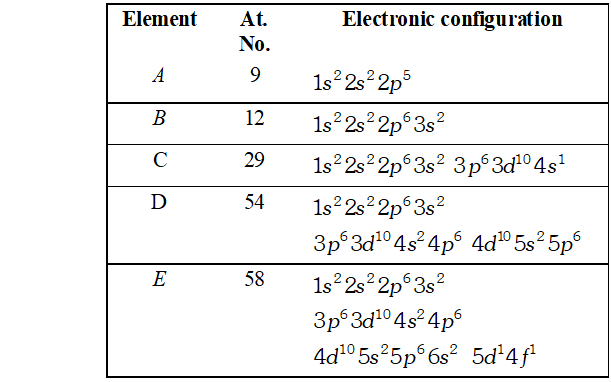(ii) Element$A$receives the last electron in$2 p-$orbital, therefore, it belongs to$p$-block elements and its group number$=10+\mathrm{No.}$of electrons in the valence shell$=10+7=17 .$Further the period of the element$=$No. of the principal quantum number of the valence shell$=2^{\mathrm{nd}}$Element$B$receives the last electron in$3 s$-orbital, therefore, it belongs to the$s$-block elements and its group number$=$No. of electrons in the valence shell$=2 .$Further the period of the element$=$No. of the principal quantum number of the valence shell$=3^{\text {rd }}$Element$C$receives the last electron in the$3 d$-orbital, therefore, it belongs to$d$block elements and its group number$=$No. of electrons in the penultimate shell and valence shell$=10+1=11 .$Further, the period of the element$=$No. of principal quantum number of the valence shell$=4^{\text {th }}$Element D receives its last electron in the 5 p-orbital, therefore, it belongs to$p$-block elements and its group number$=10+\mathrm{No.}$of electrons in the valence shell$=10+8=18 .$Further, the period of the element$=$No. of the principal quantum number of valence shell$=5^{\text {th }}$Element$E$receives its last electron in the$4 f$-orbital, therefore, it belongs to$f$-block elements. It may be noted here that the filling of 4 f-orbital occurs only when one electron has already entered$5 d$-orbital. Therefore, element$E-$belongs to$f-$block elements and not to$d$-block elements. since it belongs to lanthanide series therefore, as such it does not have any group number of its own but is usually considered to lie in group$3 .$However, its period$=$No. of the principal quantum number of the valence shell$=6^{\text {th }}$(iii) Elements$A$and$B$are representative elements since their last electron enters$p-$and$s$-orbital respectively. Elements$C$is a transition element since it receives its last electron in the$d$- orbital. Element$D$is a$p$-block element with completely filled$s$- and$p$-orbitals of the valence shell. Such a type of$p-$block element is called a noble gas. Element$E$is an inner transition element since it receives its last electron in the f-orbital. Q. Predict the formula of the stable binary compounds that would be formed by the following pairs of elements : (i) Lithium and Oxygen (ii) Magnesium and Nitrogen (iii) Aluminium and Iodine (iv) Silicon and Oxygen (v) Phosphorus and Fluorine (vi) Element 71 and Fluorine. [NCERT] Ans. (i) Lithium belongs to group 1 with a valence of 1 while oxygen belongs to group 16 with a valence of$2 .$Hence, the formula of the compound is$L i_{2} O$. (ii) Magnesium belongs to group 2 with a valence of 2 while nitrogen belongs to group 5 with a valence of 3. Hence, the formula of compound is$M g_{3} N_{2}$(iii) Aluminium belongs to group 13 with a valence of 3 and iodine belongs to group 17 with a valence of$1 .$Hence the formula of the compound is$A l l_{3}$(iv) Silicon belongs to group 14 with valence of 4 and oxygen belongs to group 16 with a valence of$2 .$Hence, the formula of the compound is$S i O_{2}$(v) Phosphorus belongs to group 15 and it has valence 3 or$5,$while fluorine belongs to group 17 with a valence of 1 Hence the formula of the compound may be$P F_{3}$or$P F_{5} .$(vi) Element with atomic number 71 is a lanthanoid called Lutetium$(L u),$its common value is$3 .$Fluorine is a group 17 (halogen) element with a valence of$1 .$Therefore, the formula of the compound formed would be$L u F_{3}$(Lutetium fluoride). Q. Describe the theory associated with the radius of an atom as it (i) gains electron (ii) loses electron. [NCERT] Ans. (i) Gain of electron. When a neutral atom gains one electron to form an anion, its radius increases. The reason being that the number of electrons in the anion increases while its nuclear charge remains the same as the parent atom. Since the same nuclear charge now attracts greater number of electrons, therefore, force of attraction of the nucleus on the electrons of all the shells decreases (i.e., effective nuclear charge decreases) and hence the electron cloud expands. In other words, the distance between the centre of the nucleus and the last shell that contains electrons increases thereby increasing the ionic radius. Thus,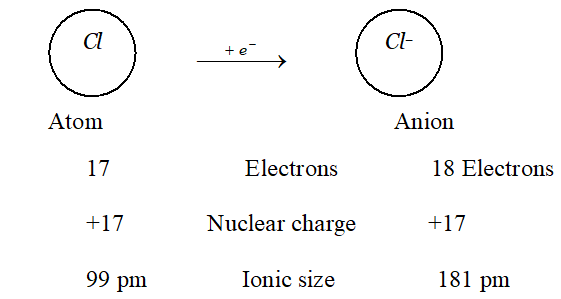(ii) Loss of electrons. When a neutral atom loses one electron to form a cation, its atomic radius decreases. The reason being that the number of electrons in the cation decreases while its nuclear charge remains the same as the parent atom. Since the same nuclear charge now attracts lesser number of electrons, therefore, the force of attraction of the nucleus on the electrons of all the shells-increases (i.e., effective nuclear charges increases) and hence the size of cation decreases. Thus,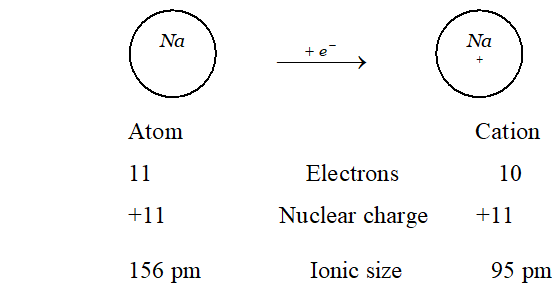Q. The first ionization enthalpy values (in$k J$mol$^{-1}$) of group 13 elements are :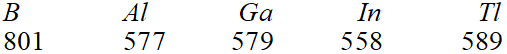How will you explain this deviation from the general trend? [NCERT] Ans. On moving the group 13 from$B$to$A l,$the ionization enthalpy decreases as expected due to an increases in atomic size and screening effect which outweigh the effect of increased nuclear charge. However,$\Delta_{i} H$of$G a$is only slightly higher$\left(2 k J m o l^{-1}\right)$than that of$A l$while that of$T i$is much higher than those of$A l, G a$and$I n .$These deviations can be explained as follows: Al follows immediately after$s$-block elements while$G a$and In follows after$d$-block elements and$T i$after$d$- and$f$- block elements. These extra$d-$and$f-$electrons do not shield (or screen) the outer shell-electrons from the nucleus very effectively. As a result, the valence electrons remain more tightly held by the nucleus and hence larger amount of energy is needed for their removal. This explains why$G a$has higher ionization enthalpy than$A l .$Further on moving down the group from$G a$to$I n,$the increased shielding effect (due to presence of additional$4 d$-electrons ) outweighs the effect of increased nuclear charge$(49-31=18$units) and hence the$\Delta_{i} H_{1}$of$\operatorname{In}$is lower than that of the$G a .$Thereafter, the effect of increased nuclear charge$(81-49=32$units ) outweigh the shielding effect due to the presence of additional 4$f$and$5 d$electrons and hence the of$T l$is higher than that of In. Q. The first$\left(\Delta_{i} H_{1}\right)$and the$\left(\Delta_{i} H_{2}\right)$ionization enthalpies$\left(\text { in } k J m o l^{-1}\right)$and the$\left(\Delta_{e g} H\right)$electron gain enthalpy (in$k J$mol$^{-1}$) of a few elements are given below: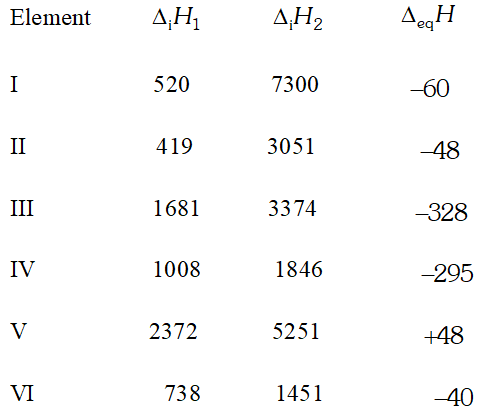Which of the above element is likely to be : (i) The least reactive metal (ii) The most reactive metal (iii) The most reactive non-metal (iv) The least reactive non-metal (v) The metal which can form a stable binary halide of the formula$M X_{2}(X=\text { halogen })$(vi) The metal which can form predominantly stable covalent halide of the formula$M X(X=\text { halogen }) ?$[NCERT] Ans. (i) The element$V$has highest first ionization enthalpy$\left(\Delta_{i} H_{1}\right)$and positive electron gain enthalpy$\left(\Delta_{e g} H\right)$and hence it is the least reactive element. since inert gases have positive$\Delta_{e g} H,$therefore, the element-V must be inert gas. The values of$\Delta_{i} H_{1}, \Delta_{i} H_{2}$and$\Delta_{e g} H,$match that of$H e .$(ii) The element II which has the least first ionization enthalpy and a low negative electron gain enthalpy is the most reactive metal. The values of and match that of$K$(potassium). (iii) The element III which has high first ionization enthalpy and a very high negative electrons gain enthalpy is the most reactive non-metal. The values of and match that of (fluorine). (iv) The element IV has a high negative electron gain enthalpy but not so high first ionization enthalpy. Therefore, it is the least reactive non-metal. the values of and match that of$I$(Iodine). (v) The element VIhas low first ionization enthalpy but higher than that of alkalimetals. Therefore, it appears that the element is an alkaline each metal and hence will form binary halide of the formula (where$X=$halogen). The values of and match that of (magnesium).$M g$(vi) The element I has low first ionization but a very high second ionization enthalpy, therefore, it must be an alkali metal. since the metal forms a predominary stable covalent halide of the formula$(X=$halogen), therefore, the alkali metal must be least reactive. athe values of and match that of Li (lithium). Q. What do you understand by isoelectronic species? Name the species that will be isoelectronic with each of the following atoms or ions.$\begin{array}{llll}{\text { (i) } F^{-}} & {\text {(ii) } A r} & {\text { (iii) } M g^{2+}} & {\text { (iv) } R b^{+}}\end{array}$[NCERT] Ans. Ions of different elements which have the same number of electrons but different magnitude of the nuclear charge are called isoelectronic ions. (i)$\quad F^{-}$has$10(9+1)$electrons. Therefore, the species nitride ion,$N^{3-}(7+3) ;$oxide ion;$O^{2-}(8+2),$neon,$N e(10+0)$sodium ion,$N a^{+}(11-1) ;$magnesium ion,$M g^{2+}(12-2)$aluminium ion,$A l^{3+}(13-3)$etc. each one of which contain 10 electrons, are isoelectronic with it. (ii)$\quad$Ar has 18 electrons. Therefore, the species phosphate ion,$P^{3-}(15+3),$sulphide ion;$S^{-2}(16+2) ;$chloride ion;$\mathrm{Cl}^{-}(17+1),$potassium ion,$K^{+}(19-1),$Calcium ion,$\mathrm{Ca}^{2+}(20-2),$etc. each one of which contain 18 electrons, are isoelectronic with it. (iii)$M g^{2+}$has$10(12-2)$electrons, therefore, the species$N^{3-}, O^{2-}, F^{-}, N e, N a^{+}, A l^{3+}$etc. each one of which contains 10 electrons, are isoelectronic with it. (iv)$\quad R b^{+}$has$36(37-1)$electrons. Therefore, the species bromide ion,$B r^{-}(35+1),$krypton,$K r(36+0)$and strontium$S r^{2+}(38-2)\$ each one of which has 36 electrons, are isoelectronic with it.

Arya
March 9, 2021, 3:25 p.m.
Good content
Monika
Dec. 10, 2020, 4:36 p.m.